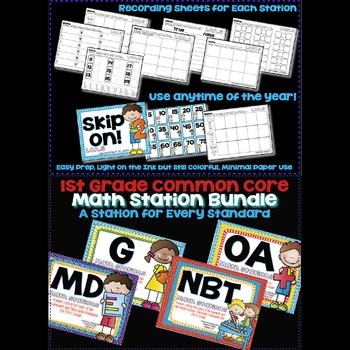# First Grade Common Core Math Stations for the Year!Subject
Resource Type
File Type
Zip (85 MB|140 pages)
Standards
\$20.00
List Price:
\$36.00
You Save:
\$16.00
\$20.00
List Price:
\$36.00
You Save:
\$16.00
• Product Description
• Standards
This bundle includes 1 math station for each of the first grade common core math standards!

There are 24 stations in all... priced at less than \$1 a station!

~These stations can be used anytime of the year regardless of season or your curriculum.
~Every station includes a recording sheet so that you can check for understanding and completion of the activity.
~The cover sheet and recording sheet of every station includes an "I CAN" statement and the standard number. This is great for teacher evaluation purposes and standards based report cards.
~These are designed to be "light" on color while still being visually engaging.
~Each station is also included in black and white for those who prefer not to print in color.

Check out a FREE SAMPLE BY CLICKING HERE!

OA Standards:
Solve for Three (1.OA.2)
Switch it Up (Commutative 1.OA.3)
Match 3 Up (Associative 1.OA.3)
Fact Family Fun (1.OA.4)
Skip On (1.OA.5)
True or False (1.OA.7)
Fix It Up (1.OA.8)

NBT Standards:
Fill It In (1.NBT.1)
Matching 10's (1.NBT.2a)
11 to 19 (1.NBT.2b)
Comparing Numbers (1.NBT.3)
10 More & 10 Less (1.NBT.5)
Subtract with 10 (1.NBT.6)

Measurement & Data Standards:
Shortest & Longest (1.MD.1)
Measure It Up (1.MD.2)
It's Time (1.MD.3)
Graph It! (1.MD.4)

Geometry Standards:
Sorting Shapes (1.G.1)
Make a New Shape (1.G.2)
Fraction Action (1.G.3)

Partition circles and rectangles into two and four equal shares, describe the shares using the words halves, fourths, and quarters, and use the phrases half of, fourth of, and quarter of. Describe the whole as two of, or four of the shares. Understand for these examples that decomposing into more equal shares creates smaller shares.
Compose two-dimensional shapes (rectangles, squares, trapezoids, triangles, half-circles, and quarter-circles) or three-dimensional shapes (cubes, right rectangular prisms, right circular cones, and right circular cylinders) to create a composite shape, and compose new shapes from the composite shape.
Distinguish between defining attributes (e.g., triangles are closed and three-sided) versus non-defining attributes (e.g., color, orientation, overall size); build and draw shapes to possess defining attributes.
Determine the unknown whole number in an addition or subtraction equation relating three whole numbers. For example, determine the unknown number that makes the equation true in each of the equations 8 + ? = 11, 5 = ▯ - 3, 6 + 6 = ▯.
Understand the meaning of the equal sign, and determine if equations involving addition and subtraction are true or false. For example, which of the following equations are true and which are false? 6 = 6, 7 = 8 - 1, 5 + 2 = 2 + 5, 4 + 1 = 5 + 2.
Total Pages
140 pages